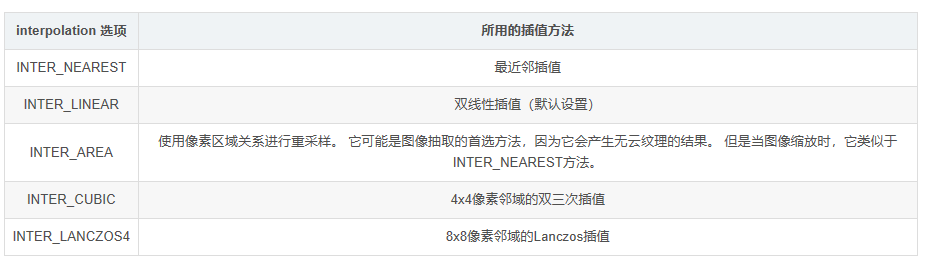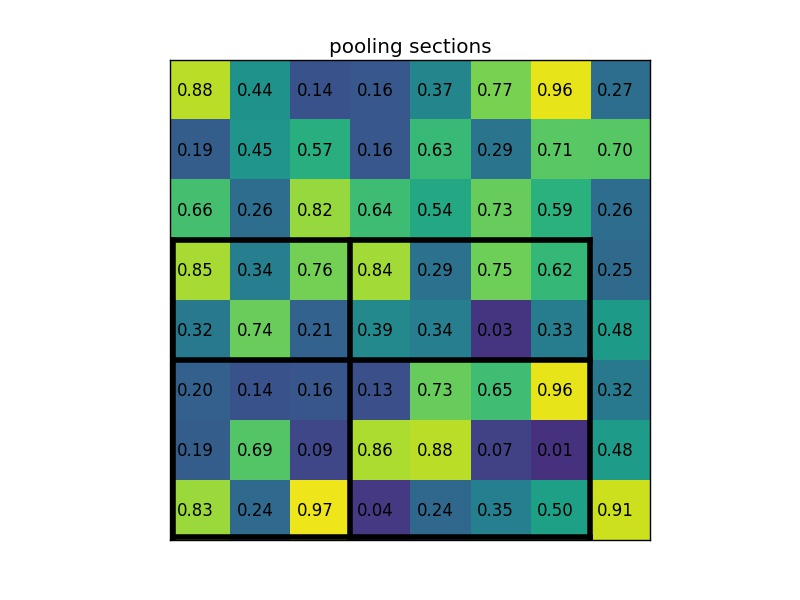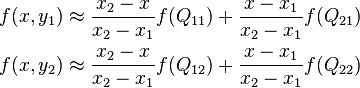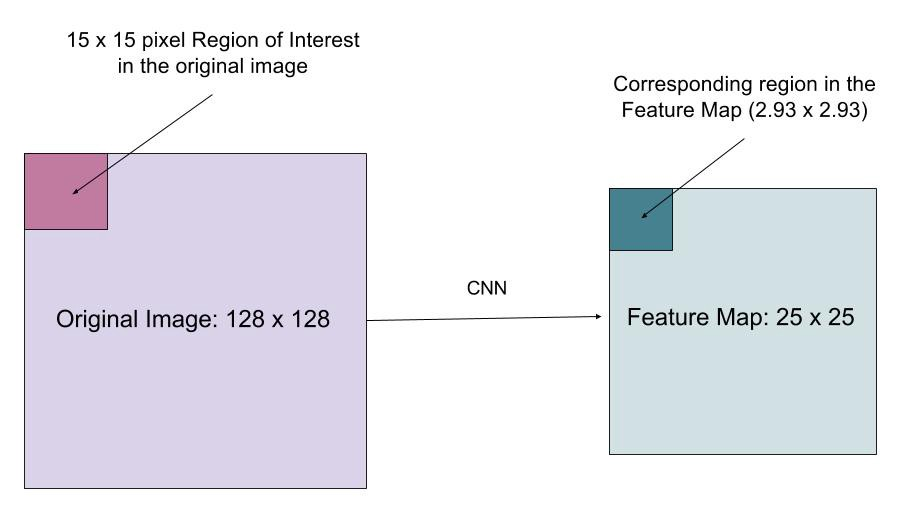2019-07-19 15:54:31 qq_29507011 阅读数 189
• ###### 学习OpenCV3.2+QT5+ffmpeg实战开发视频编辑器视频教程

OpenCV3.2+QT5+ffmpeg实战开发视频编辑器视频培训课程概况：教程中会讲解到基于opencv视频和摄像机录制、播放和播放进度控制，多视频图像合并、多视频图像融合、剪切、视频亮度、对比度、尺寸（近邻插值（手动实现），双线性插值，图像金字塔）、颜色格式（灰度图，二值化（阈值）），旋转镜像，视频裁剪（ROI），视频水印（ROI+weight），导出处理后的视频（包含音频，使用ffmpeg工具对音频进行抽取、剪切和终于opencv处理的视频合并）。

19532 人正在学习 去看看 夏曹俊

cv2.resize(img, (0, 0), fx=倍数, fy=倍数, interpolation=cv2.INTER_NEAREST)

x0 = x1/scale y0 = y1/scale x = int(x0) y = int(y0) u = x0-x v = y0-y
f(y1,x1) = (1-v)(1-u)f(y,x)+(1-v)uf(y,x+1)+v(1-u)f(y+1,x)+uvf(y+1,x+1)

cv2.resize(img, (0, 0), fx=倍数, fy=倍数, interpolation=cv2.INTER_LINEAR)

cv2.resize(img, (0, 0), fx=倍数, fy=倍数, interpolation=cv2.INTER_AREA)

cv2.resize(img, (0, 0), fx=倍数, fy=倍数, interpolation=cv2.INTER_CUBIC)

cv2.resize(img, (0, 0), fx=倍数, fy=倍数, interpolation=cv2.INTER_LANCZOS4)

``````import numpy as np
import cv2

path = 'test.jpg'

def near(image,scale):
hight,width = image.shape
img = np.zeros((hight*scale,width*scale))
for i in range(img.shape-4):
for j in range(img.shape-4):
x = round(i/scale)
y = round(j/scale)
img[i,j] = image[x,y]

return img

img = near(image,4)
cv2.imshow('test',img.astype(np.uint8))
cv2.waitKey(0)
cv2.destroyWindow()
````````````def double_insert(image,scale):
hight,width = image.shape
h = hight*scale
w = width*scale
img = np.zeros((h,w))
for i in range(h-4):
for j in range(w-4):
y = int(i/scale)
x = int(j/scale)
img[i,j] = (y+1-i/scale)*(x+1-j/scale)*image[y,x]+(y+1-i/scale)*(j/scale-x)*image[y,x+1]+(i/scale-y)*(x+1-j/scale)*image[y+1,x]+(i/scale-y)*(j/scale-x)*image[y+1,x+1]
return img
``````2018-05-14 17:07:29 qq_35447659 阅读数 301
• ###### 学习OpenCV3.2+QT5+ffmpeg实战开发视频编辑器视频教程

OpenCV3.2+QT5+ffmpeg实战开发视频编辑器视频培训课程概况：教程中会讲解到基于opencv视频和摄像机录制、播放和播放进度控制，多视频图像合并、多视频图像融合、剪切、视频亮度、对比度、尺寸（近邻插值（手动实现），双线性插值，图像金字塔）、颜色格式（灰度图，二值化（阈值）），旋转镜像，视频裁剪（ROI），视频水印（ROI+weight），导出处理后的视频（包含音频，使用ffmpeg工具对音频进行抽取、剪切和终于opencv处理的视频合并）。

19532 人正在学习 去看看 夏曹俊

1.最近邻插值

```import cv2
import numpy as np
import time
from traitlets.config.application import catch_config_error
def function(img,dstHeight,dstWidth):
height,width,channels =img.shape
emptyImage=np.zeros((dstHeight,dstWidth,channels),np.uint8)
sh=dstHeight/height
sw=dstWidth/width
for i in range(dstHeight):
for j in range(dstWidth):
x=min(round(i/sh), height-1)
y=min(round(j/sw), width-1)
emptyImage[i,j]=img[x,y]
return emptyImage
if __name__ == '__main__':
start = time.time()
dstHeight=200
dstWidth=200
img_out=function(img_in,dstHeight,dstWidth)
print ('cost %f seconds' % (time.time() - start))
cv2.imshow('src_image', img_in)
cv2.imshow('dst_image', img_out)
cv2.waitKey()  ```

2.双线性插值

```# coding=utf-8
import cv2
import numpy as np
import time
def resize(src, new_size):
dst_w, dst_h = new_size # 目标图像宽高
src_h, src_w = src.shape[:2] # 源图像宽高
if src_h == dst_h and src_w == dst_w:
return src.copy()
scale_x = float(src_w) / dst_w # x缩放比例
scale_y = float(src_h) / dst_h # y缩放比例
# 遍历目标图像，插值
dst = np.zeros((dst_h, dst_w, 3), dtype=np.uint8)
for n in range(3): # 对channel循环
for dst_y in range(dst_h): # 对height循环
for dst_x in range(dst_w): # 对width循环
# 目标在源上的坐标
src_x = (dst_x + 0.5) * scale_x - 0.5  #定位像素点：中心对齐
src_y = (dst_y + 0.5) * scale_y - 0.5
# 计算在源图上四个近邻点的位置
src_x_0 = int(np.floor(src_x))
src_y_0 = int(np.floor(src_y))
src_x_1 = min(src_x_0 + 1, src_w - 1)
src_y_1 = min(src_y_0 + 1, src_h - 1)
# 双线性插值
# 设(x,y)为插值点在源图像的坐标，待计算插值为z
# 首先x方向上插值：相邻两点为(x0,y0)、(x1,y0)，像素值分别为z0=f(x0,y0)、z1=f(x1,y0)，从而有公式：z−z0x−x0=z1−z0x1−x0，从而插值z=x1−xx1−x0z0+x−x0x1−x0z1
#
# 然后y方向上插值：以上得到的上方插值z
# 记为ztop，同理可得下方插值为zbot，那么最后插值为：Z=y1−yy1−y0ztop+y−y0y1−y0zbot
value0 = (src_x_1 - src_x) * src[src_y_0, src_x_0, n] + (src_x - src_x_0) * src[src_y_0, src_x_1, n]
value1 = (src_x_1 - src_x) * src[src_y_1, src_x_0, n] + (src_x - src_x_0) * src[src_y_1, src_x_1, n]
dst[dst_y, dst_x, n] = int((src_y_1 - src_y) * value0 + (src_y - src_y_0) * value1)
return dst

if __name__ == '__main__':
start = time.time()
img_out = cv2.resize(img_in, (600,600))
print ('cost %f seconds' % (time.time() - start))

cv2.imshow('src_image', img_in)
cv2.imshow('dst_image', img_out)
cv2.waitKey()```

https://blog.csdn.net/smf0504/article/details/51303962

https://blog.csdn.net/xbinworld/article/details/65660665

https://blog.csdn.net/qq_29058565/article/details/52769497

2015-08-20 16:18:06 zhangchen1003 阅读数 1595
• ###### 学习OpenCV3.2+QT5+ffmpeg实战开发视频编辑器视频教程

OpenCV3.2+QT5+ffmpeg实战开发视频编辑器视频培训课程概况：教程中会讲解到基于opencv视频和摄像机录制、播放和播放进度控制，多视频图像合并、多视频图像融合、剪切、视频亮度、对比度、尺寸（近邻插值（手动实现），双线性插值，图像金字塔）、颜色格式（灰度图，二值化（阈值）），旋转镜像，视频裁剪（ROI），视频水印（ROI+weight），导出处理后的视频（包含音频，使用ffmpeg工具对音频进行抽取、剪切和终于opencv处理的视频合并）。

19532 人正在学习 去看看 夏曹俊

1.1怎么理解图像插值

1.2 常用的图像插值方法

3.1 图像的缩放
matlab中可以用imresize函数对图像进行缩放，使用形式：
imresize（A，n）A为图像，n为放大倍数。
imresize（A，[n,m]）把原有的图像放大为行列分别为n,m的图像。

1.最近邻插值法：是imreseze默认的方法，
2.双线性插值方法：格式：imresize（A，n，’bilinear’）.
3.双立方插值方法：格式：imresize（A，n，’bicubic’）.

``````clear;
clc;
close all;
f=rgb2gray(f);
[m,n]=size(f);
X=1:m;
Y=1:n;
[X,Y]=meshgrid(X,Y);%生成网格矩阵
X1=1:0.5:m;
Y1=1:0.5:n;
[X1,Y1]=meshgrid(X1,Y1);生成网格矩阵
J=interp2(X,Y,f,X1,Y1,'nearest');进行插值运算
figure,imshow(f)
figure,
imshow(J)``````

3.2图像的旋转

Angle：旋转地角度，
Method：为插值的方法，nearest、bilinear、bicubic
Bbox：loose和crop

3.3图像的裁剪

imcrop（A，[80,120,100,50]）;
[80,120,100,50]的含义是，以图像的（80，,10）点位裁剪矩形的左上角坐标，裁剪的宽度是100，高度是50。

3.4图像的几何变换

imtransform（A,T）
A：是要变换的图像
T：是由makeform函数产生的变换结构.

affine：仿射变换形式。
projective:投影变换形式；
cusyom：自定义函数变换；
box：利用函数中的另外参数产生仿射变换结构；
composite：该参数是实现多次调用tformfwd功能；

``````I=imread('peppers.png');
Ta = maketform('affine', ...
[cosd(30) -sind(30) 0; sind(30) cosd(30) 0; 0 0 1]');% 创建旋转参数结构体
Ia = imtransform(I,Ta); %实现图像旋转
Tb = maketform('affine',[5 0 0; 0 10.5 0; 0 0 1]'); %创建缩放参数结构体
Ib = imtransform(I,Tb);%实现图像缩放
``````

2014-10-29 08:36:17 phoenixtree7 阅读数 533
• ###### 学习OpenCV3.2+QT5+ffmpeg实战开发视频编辑器视频教程

OpenCV3.2+QT5+ffmpeg实战开发视频编辑器视频培训课程概况：教程中会讲解到基于opencv视频和摄像机录制、播放和播放进度控制，多视频图像合并、多视频图像融合、剪切、视频亮度、对比度、尺寸（近邻插值（手动实现），双线性插值，图像金字塔）、颜色格式（灰度图，二值化（阈值）），旋转镜像，视频裁剪（ROI），视频水印（ROI+weight），导出处理后的视频（包含音频，使用ffmpeg工具对音频进行抽取、剪切和终于opencv处理的视频合并）。

19532 人正在学习 去看看 夏曹俊

[转自] http://blog.csdn.net/yangtrees/article/details/8896531

1、最邻近元法

这是最简单的一种插值方法，不需要计算，在待求象素的四邻象素中，将距离待求象素最近的邻象素灰度赋给待求象素。设i+u, j+v(i, j为正整数， u, v为大于零小于1的小数，下同)为待求象素坐标，则待求象素灰度的值 f(i+u, j+v)　如下图所示：2、双线性内插法f(i, j+v) = [f(i, j+1) - f(i, j)] * v + f(i, j)

f(i+1, j+v) = [f(i+1, j+1) - f(i+1, j)] * v + f(i+1, j)

f(i+u, j+v) = (1-u) * (1-v) * f(i, j) + (1-u) * v * f(i, j+1) + u * (1-v) * f(i+1, j) + u * v * f(i+1, j+1)

3、三次内插法f(x, y) = f(i+u, j+v) = ABC“Inverse Distance to a Power（反距离加权插值法）”、
“Kriging（克里金插值法）”、
“Minimum Curvature（最小曲率)”、
“Modified Shepard's Method（改进谢别德法）”、
“Natural Neighbor（自然邻点插值法）”、
“Nearest Neighbor（最近邻点插值法）”、
“Polynomial Regression（多元回归法）”、
“Triangulation with Linear Interpolation（线性插值三角网法）”、
“Moving Average（移动平均法）”、
“Local Polynomial（局部多项式法）”

1、距离倒数乘方法

2、克里金法

3、最小曲率法

4、多元回归法

5、径向基本函数法

6、谢别德法

7、三角网/线形插值法

8.自然邻点插值法

9.最近邻点插值法

Reference

http://blog.csdn.net/coy_wang/article/details/5027872

http://blog.sina.com.cn/s/blog_6e51df7f0100vb4b.html

2019-01-21 16:03:41 xjp_xujiping 阅读数 184
• ###### 学习OpenCV3.2+QT5+ffmpeg实战开发视频编辑器视频教程

OpenCV3.2+QT5+ffmpeg实战开发视频编辑器视频培训课程概况：教程中会讲解到基于opencv视频和摄像机录制、播放和播放进度控制，多视频图像合并、多视频图像融合、剪切、视频亮度、对比度、尺寸（近邻插值（手动实现），双线性插值，图像金字塔）、颜色格式（灰度图，二值化（阈值）），旋转镜像，视频裁剪（ROI），视频水印（ROI+weight），导出处理后的视频（包含音频，使用ffmpeg工具对音频进行抽取、剪切和终于opencv处理的视频合并）。

19532 人正在学习 去看看 夏曹俊

## Syntax

cv2.resize(src, dsize[, dst[, fx[, fy[, interpolation]]]]) → dst## INTER_NEAREST | 最近邻插值## INTER_LINEAR | 双线性插值（默认设置）``````srcX = dstX* (srcWidth/dstWidth)
srcY = dstY * (srcHeight/dstHeight)``````

来计算目标像素在源图像中的位置，这里计算的srcX和srcY一般都是浮点数，比如 `f（1.2, 3.4）`这个像素点是虚拟存在的，先找到与它临近的四个实际存在的像素点

``````（1，3） （2，3）
（1，4） （2，4）``````

``f(i+u,j+v) = (1-u)(1-v)f(i,j) + (1-u)vf(i,j+1) + u(1-v)f(i+1,j) + uvf(i+1,j+1) ``INTER_AREA |  使用像素区域关系进行重采样。

INTER_CUBIC  | 4x4像素邻域的双三次插值

INTER_LANCZOS4 |  8x8像素邻域的Lanczos插值

## Code

``````# coding=utf-8

import cv2
"""
INTER_NEAREST | 最近邻插值
INTER_LINEAR | 双线性插值（默认设置）
INTER_AREA |  使用像素区域关系进行重采样
INTER_CUBIC  | 4x4像素邻域的双三次插值
INTER_LANCZOS4 |  8x8像素邻域的Lanczos插值
"""

if __name__ == '__main__':
height, width = img.shape[:2]

# 缩小图像
size = (int(width*0.8), int(height*0.7))
shrink_NEAREST = cv2.resize(img, size, interpolation=cv2.INTER_NEAREST)
shrink_LINEAR = cv2.resize(img, size, interpolation=cv2.INTER_LINEAR)
shrink_AREA = cv2.resize(img, size, interpolation=cv2.INTER_AREA)
shrink_CUBIC = cv2.resize(img, size, interpolation=cv2.INTER_CUBIC)
shrink_LANCZOS4 = cv2.resize(img, size, interpolation=cv2.INTER_LANCZOS4)

# 放大图像
fx = 1.2
fy = 1.1
enlarge_NEAREST = cv2.resize(img, (0, 0), fx=fx, fy=fy, interpolation=cv2.INTER_NEAREST)
enlarge_LINEAR = cv2.resize(img, (0, 0), fx=fx, fy=fy, interpolation=cv2.INTER_LINEAR)
enlarge_AREA = cv2.resize(img, (0, 0), fx=fx, fy=fy, interpolation=cv2.INTER_AREA)
enlarge_CUBIC = cv2.resize(img, (0, 0), fx=fx, fy=fy, interpolation=cv2.INTER_CUBIC)
enlarge_LANCZOS4 = cv2.resize(img, (0, 0), fx=fx, fy=fy, interpolation=cv2.INTER_LANCZOS4)

# 保存图像
cv2.imwrite("shrink_NEAREST.jpg", shrink_NEAREST)
cv2.imwrite("shrink_LINEAR.jpg", shrink_LINEAR)
cv2.imwrite("shrink_AREA.jpg", shrink_AREA)
cv2.imwrite("shrink_CUBIC.jpg", shrink_CUBIC)
cv2.imwrite("shrink_LANCZOS4.jpg", shrink_LANCZOS4)

cv2.imwrite("enlarge_NEAREST.jpg", enlarge_NEAREST)
cv2.imwrite("enlarge_LINEAR.jpg", enlarge_LINEAR)
cv2.imwrite("enlarge_AREA.jpg", enlarge_AREA)
cv2.imwrite("enlarge_CUBIC.jpg", enlarge_CUBIC)
cv2.imwrite("enlarge_LANCZOS4.jpg", enlarge_LANCZOS4)
---------------------

## Demo### 利用插值缩小4x4像素邻域的双三次插值：8x8像素邻域的Lanczos插值：### 利用插值放大4x4像素邻域的双三次插值：8x8像素邻域的Lanczos插值：---------------------